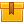# Wye and Delta Connections

## Prerequisites

The lesson requires a fundamental knowledge of electrical theory and technology. Basic knowledge of 3-phase circuits is recommended.

## Description

The tenth lesson in the iKNOW Electrical Theory for Troubleshooters Library, this lesson discusses Wye and Delta configurations and explains the relationship between phase and line voltages in various connections, and demonstrates the application of the formula that shows this relationship.

## Objectives

Describe the relationship between phase and line voltages in a 3-wire wye connection. Describe the relationship between phase and line voltages in a 4-wire wye connection. Describe the relationship between phase and line voltages in a 4-wire wye connection. Describe the relationship between phase and line currents in a delta connection. Calculate power in a 3-phase load. Apply the formula that shows the relationship between phase and line currents in a 3-wire wye connection. Apply the formula that shows the relationship between phase voltage and single-phase load voltage in a 4-wire wye connection. Describe the the relationship between phase and line voltages in a delta connection.

## ContentThe tenth lesson in the iKNOW™ Electrical Theory for Troubleshooters
Library, this lesson discusses Wye and Delta configurations and explains
the relationship between phase and line voltages in various
connections, and demonstrates the application of the formula that shows
this relationship.

•Wye Delta Connections

0
0 Reviews